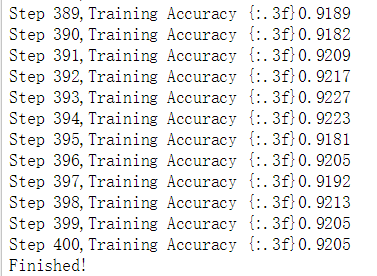一 、MNIST手写数字数据集介绍

MNIST手写数字数据集来源于是美国国家标准与技术研究所，是著名的公开数据集之一，通常这个数据集都会被作为深度学习的入门案例。数据集中的数字图片是由250个不同职业的人纯手写绘制，数据集获取的网址为：http://yann.lecun.com/exdb/mnist/。（下载后需解压）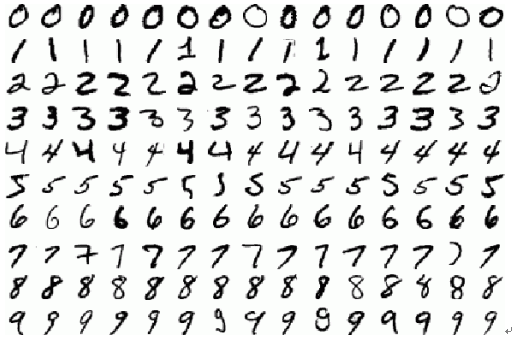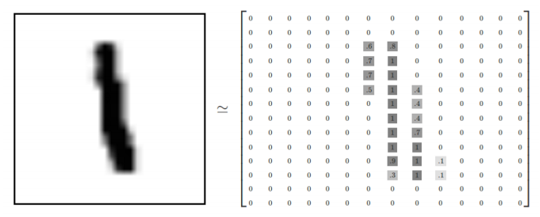二 、网络结构的设计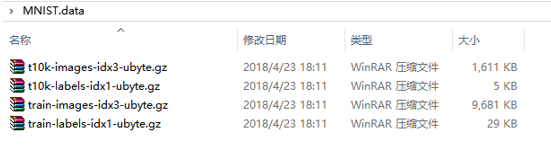调用神经网络

result = neural_network(x)

预测类别

prediction = tf.nn.softmax(result)

平方差损失函数

loss = tf.reduce_mean(tf.square(y-prediction))

预测类标

correct_pred = tf.equal(tf.argmax(y,1),tf.argmax(prediction,1))

计算准确率

accuracy = tf.reduce_mean(tf.cast(correct_pred,tf.float32))

初始化变量

init = tf.global_variables_initializer() 如程序6所示：首先使用softmax函数对结果进行预测，然后选择平方差损失函数计算出loss，再使用梯度下降法的优化方法对loss进行最小化（梯度下降法的学习率设置为0.2）。接着使用argmax函数返回最大的值所在的位置，再使用equal函数与正确的类标进行比较，返回一个bool值，代表预测正确或错误的类标；最后使用cast函数把bool类型的预测结果转换为float类型（True转换为1，False转换为0），并对所有预测结果统计求平均值，算出最后的准确率。要注意：最后一定不要忘了对程序中的所有变量进行初始化。 最后一步，我们启动Tensorflow默认会话，执行上述过程。代码如下所示： 程序7： step_num=400 with tf.Session() as sess: sess.run(init) for step in range(step_num+1): for batch in range(batch_num): batch_x,batch_y = mnist_data.train.next_batch(batch_size) sess.run(train_step,feed_dict={x:batch_x,y:batch_y}) acc = sess.run(accuracy,feed_dict={x:mnist_data.test.images,y:mnist_data.test.labels}) print("Step " + str(step) + ",Training Accuracy "+ "{:.3f}" + str(acc)) print("Finished!") 上述程序定义了MNIST数据集的运行阶段，首先我们定义迭代的周期数，往往开始的时候准确率会随着迭代次数快速提高，但渐渐地随着迭代次数的增加，准确率提升的幅度会越来越小。而对于每一轮的迭代过程，我们用不同批次的图片进行训练，每次训练100张图片，每次训练的图片数据和对应的标签分别保存在 batch_x、batch_y中，接着再用run方法执行这个迭代过程，并使用feed_dict的字典结构填充每次的训练数据。循环往复上述过程，直到最后一轮的训练结束。 最后我们利用测试集的数据检验训练的准确率，feed_dict填充的数据分别是测试集的图片数据和测试集图片对应的标签。输出结果迭代次数和准确率，完成训练过程。我们截取400次的训练结果，如下图所示：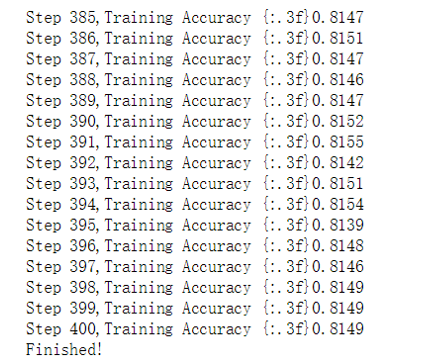预测类别

prediction = tf.nn.softmax(result)

交叉熵损失函数

loss = tf.reduce_mean(tf.nn.softmax_cross_entropy_with_logits(labels=y,logits=prediction))

预测类标

correct_pred = tf.equal(tf.argmax(y,1),tf.argmax(prediction,1))

计算准确率

accuracy = tf.reduce_mean(tf.cast(correct_pred,tf.float32)) 如程序8所示：我们把两个参数：类标y以及模型的预测值prediction，传入到交叉熵损失函数softmax_cross_entropy_with_logits中，然后对函数的输出结果求平均值，再使用梯度下降法进行优化。最终的准确率如下图所示：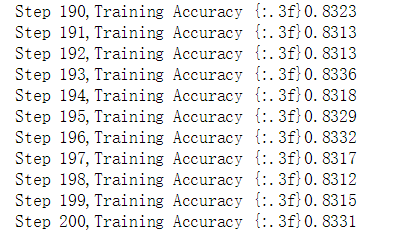train_step = tf.train.AdamOptimizer(1e-2).minimize(loss) 此外，如果你了解过拟合的概念，那么很容易可以联想到测试集准确率不高的原因，可能是因为训练过程中发生了“过拟合”的现象。所以我们可以从防止过拟合的角度出发，提高模型的准确率。我们可以采用增加数据量或是增加正则化项的方式，来缓解过拟合。这里，我们为大家介绍dropout的方式是如何缓解过拟合的。 Dropout是在每次神经网络的训练过程中，使得部分神经元工作而另外一部分神经元不工作。而测试的时候激活所有神经元，用所有的神经元进行测试。这样便可以有效的缓解过拟合，提高模型的准确率。具体代码如下所示： 程序10： def neural_network(x): hidden_layer_1 = tf.add(tf.matmul(x, weights['hidden_1']), biases['b1']) L1 = tf.nn.tanh(hidden_layer_1) dropout1 = tf.nn.dropout(L1,0.5) out_layer = tf.matmul(dropout1, weights['out']) + biases['out'] return out_layer 如程序10所示，我们在隐藏层后接了dropout，随机关掉50%的神经元，最后的测试结果如下图所示，我们发现准确率取得了显著的提高，在神经网络结构中没有添加卷积层和池化层的情况下，准确率达到了92%以上。